# Integral of cot(x)

In this post you’ll learn how to integrate the trigonometric function cot(x). But first:

What is cot(x)?

Maybe you already know it, but this is going to be really useful. The cotangent is the inverse of the tangent: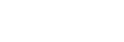This means that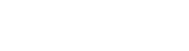Integral of cot(x)

Now we can write the integral: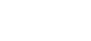Since cot(x)=cos(x)/sin(x), we have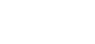We know the integral of cos(x) and the integral of sin(x), so wouldn’t it be nice to just have sin(x) or cos(x)? In order to do so, we need to get rid of sin(x) or cos(x). When you use u-substitution, the derivative of what you let be equal to u goes to the denominator, so we can’t remove sin(x) but we can get rid of cos(x). What is the function of which the derivative is cos(x)? sin(x)! This is going to be our u.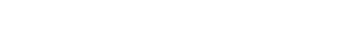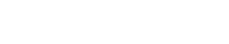So much better! And we know that this integral is equal to the natural logarithm ln(u);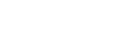Don’t write the “+C” yet because we need to undo the u-substitution, keeping in mind that u=sin(x);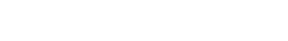This is the result. As you can see I’ve written ln|u| instead of ln(u). Both are correct, but the absolute value tells you that the argument of the logarithm can’t be negative (well, it can, but the result won’t be a real number).

Hope everything was clear! If you have any questions leave a comment and I’ll be happy to reply! And if you want, you can leave the answer to this definite integral!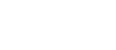Join 31 other subscribers.